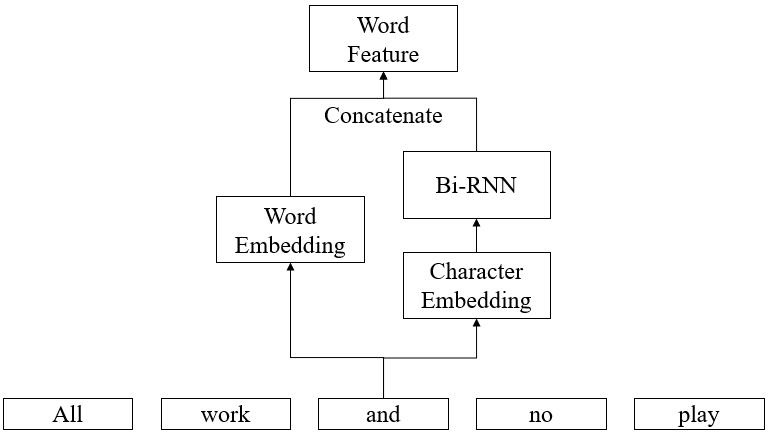# Concatenate word and character embeddings in KerasCyberZHG, updated 🕥 2022-01-22 11:33:42

# Word/Character Embeddings in Keras

## IntroductionOut-of-vocabulary words are drawbacks of word embeddings. Sometimes both word and character features are used. The characters in a word are first mapped to character embeddings, then a bidirectional recurrent neural layer is used to encode the character embeddings to a single vector. The final feature of a word is the concatenation of the word embedding and the encoded character feature.

The repository contains some functions and a wrapper class that could be used to generate the first few layers that encodes the features of words and characters.

## Install

```bash pip install keras-word-char-embd```

### Demo

There is a sentiment analysis demo in the `demo` directory. Run the following commands, then your model should have about 70% accuracy:

```bash cd demo ./get_data.sh python sentiment_analysis.py```

### Functions

This section only introduces the basic usages of the functions. For more detailed information please refer to the demo and the doc comments describing the functions in the source code.

#### `get_dicts_generator`

The function returns a closure used to generate word and character dictionaries. The closure should be invoked for all the training sentences in order to record the frequencies of each word or character. After that, setting the parameter `return_dict=True` the dictionaries would be returned.

```python from keras_wc_embd import get_dicts_generator

sentences = [ ['All', 'work', 'and', 'no', 'play'], ['makes', 'Jack', 'a', 'dull', 'boy', '.'], ] dict_generator = get_dicts_generator( word_min_freq=2, char_min_freq=2, word_ignore_case=False, char_ignore_case=False, ) for sentence in sentences: dict_generator(sentence)

word_dict, char_dict, max_word_len = dict_generator(return_dict=True) ```

You can generate dictionaries on your own, but make sure index `0` and index for `<UNK>` are preserved.

#### `get_embedding_layer`

Generate the first few layers that encodes words in a sentence:

```python from tensorflow import keras from keras_wc_embd import get_embedding_layer

inputs, embd_layer = get_embedding_layer( word_dict_len=len(word_dict), char_dict_len=len(char_dict), max_word_len=max_word_len, word_embd_dim=300, char_embd_dim=50, char_hidden_dim=150, char_hidden_layer_type='lstm', ) model = keras.models.Model(inputs=inputs, outputs=embd_layer) model.summary() ```

The output shape of `embd_layer` should be `(None, None, 600)`, which represents the batch size, the length of sentence and the length of encoded word feature.

`char_hidden_layer_type` could be `'lstm'`, `'gru'`, `'cnn'`, a Keras layer or a list of Keras layers. Remember to add `MaskedConv1D` and `MaskedFlatten` to custom objects if you are using `'cnn'`:

#### `get_batch_input`

The function is used to generate the batch inputs for the model.

```python from keras_wc_embd import get_batch_input

word_embd_input, char_embd_input = get_batch_input( sentences, max_word_len=max_word_len, word_dict=word_dict, char_dict=char_dict, ) ```

#### `get_embedding_weights_from_file`

A helper function that loads pre-trained embeddings for initializing the weights of the embedding layer. The format of the file should be similar to GloVe.

```python from keras_wc_embd import get_embedding_layer, get_embedding_weights_from_file

word_embd_weights = get_embedding_weights_from_file(word_dict, 'glove.6B.100d.txt', ignore_case=True) inputs, embd_layer = get_embedding_layer( word_dict_len=len(word_dict), char_dict_len=len(char_dict), max_word_len=max_word_len, word_embd_dim=300, char_embd_dim=50, char_hidden_dim=150, word_embd_weights=word_embd_weights, char_hidden_layer_type='lstm', ) ```

### Wrapper Class `WordCharEmbd`

There is a wrapper class that makes things easier.

```python from keras_wc_embd import WordCharEmbd

sentences = [ ['All', 'work', 'and', 'no', 'play'], ['makes', 'Jack', 'a', 'dull', 'boy', '.'], ] wc_embd = WordCharEmbd( word_min_freq=0, char_min_freq=0, word_ignore_case=False, char_ignore_case=False, ) for sentence in sentences: wc_embd.update_dicts(sentence)

inputs, embd_layer = wc_embd.get_embedding_layer() lstm_layer = keras.layers.LSTM(units=5, name='LSTM')(embd_layer) softmax_layer = keras.layers.Dense(units=2, activation='softmax', name='Softmax')(lstm_layer) model = keras.models.Model(inputs=inputs, outputs=softmax_layer) model.compile( optimizer='adam', loss=keras.losses.sparse_categorical_crossentropy, metrics=[keras.metrics.sparse_categorical_accuracy], ) model.summary()

def batch_generator(): while True: yield wc_embd.get_batch_input(sentences), np.asarray([0, 1])

model.fit_generator( generator=batch_generator(), steps_per_epoch=200, epochs=1, ) ```

## Citation

Several papers have done the same thing. Just choose the one you have seen.

## Issues

### AttributeError: Can't pickle local object 'get_dicts_generator.<locals>.get_dicts'

opened on 2019-11-19 06:20:07 by mustfkeskin

We cant pickle wc_embed objects How we can predict this repo in deployment

`AttributeError: Can't pickle local object 'get_dicts_generator.<locals>.get_dicts'`

### get_batch_input --> max_sentence_len

opened on 2019-11-18 08:41:02 by mustfkeskin

Hello

I think max sentence length must be optinal. max_sentence_len = max(map(len, sentences)

opened on 2019-10-24 07:20:22 by behrouzsalimi

in other word, how do i write batch_generator() for text generation?